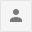# How to find the ascii value of a character in javaA computer is an electronic device that processes data according to instructions and converts it into useful information. Data or information processed from a computer is used in many tasks. If you are programming.  You need to know what is meant by computer codes. Data and instructions are given to your friends computer in machine language. Therefore, all the data whether it is numeric or non numeric has to be converted into machine language.  Coding can be defined as the way in which numeric and non-numeric data are displayed in a specific order of bits so that the computer can understand them is called coding. Different codes for displaying information in a computer  The most commonly used codes are as follows:The word ASCII stands for American Standard Code for Information Interchange. It is a standard coding scheme for displaying alphanumeric data types in computers.  ISO introduced by ISO means International standard organization.  This is a 7 bit coding scheme. The values ​​of ASCII code for different characters are different codes of A and a. Nowadays most of the computers use 8 bit ASCII code. 256 characters can be represented in 8 bit ASCII code. Data in computer is expressed in binary code.  Therefore, to represent any character in binary code, we change its value from decimal system to binary. Whatever the programming language, ASCII code is used in it. Similarly, it is also used in Java.  Let's do some practical and try to understand more ASCII.

Example:-

``````

using System;

public class AsciiValue
{
public static void Main()
{

char c = 'K';
int ascii = c;
Console.Write("The ASCII value of " +
c + " is: " + ascii);
}
}

OUTPUT
The ASCII value of e is 107``````ReplyForward